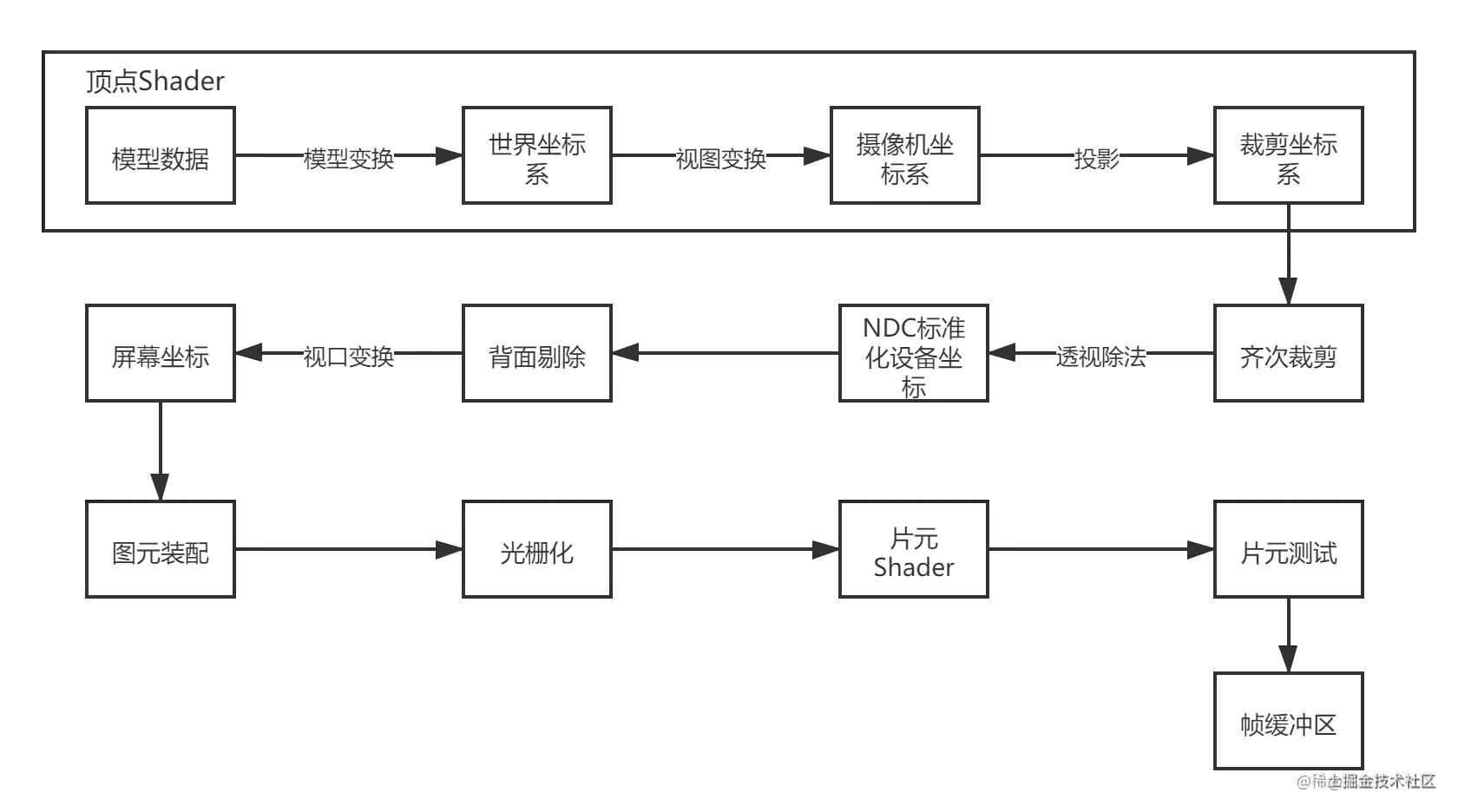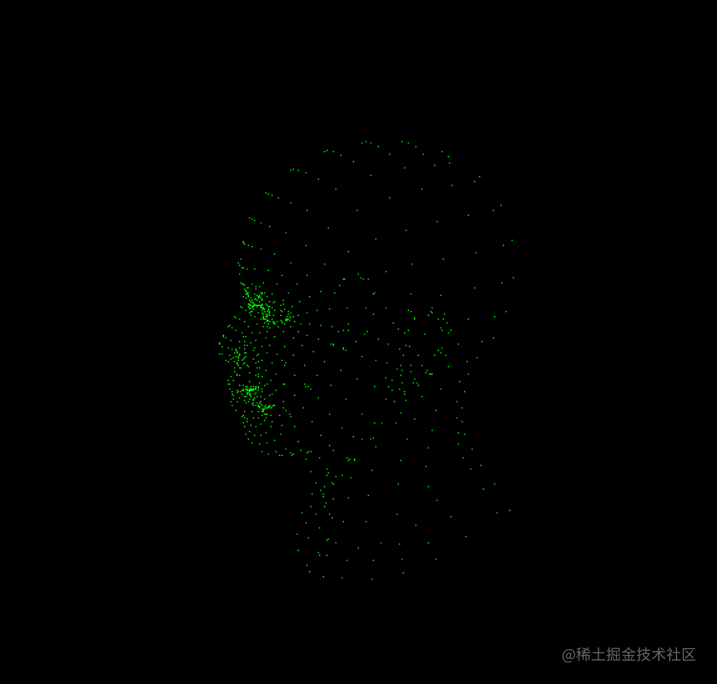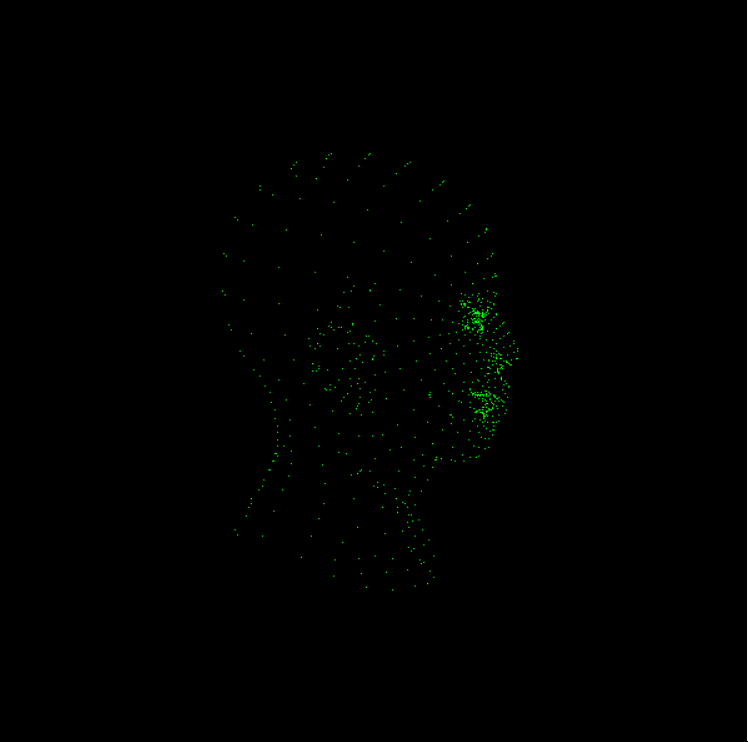# [图形学笔记系列] 实现一个简单的软光栅渲染效果-02

## 目标## 实践部分

### 裁剪坐标系

            let m = glMatrix.mat4.create()    //模型变换矩阵，这里默认单位矩阵好了
glMatrix.mat4.fromYRotation(m, Math.PI/4)  //这里我要求点坐标围绕Y轴逆时针转45°

let v = glMatrix.mat4.create()   //创建视图矩阵
let eye = glMatrix.vec3.fromValues(1, 1, 4)
let center = glMatrix.vec3.fromValues(0, 0, 0)
let up = glMatrix.vec3.fromValues(0, 1, 0)
glMatrix.mat4.lookAt(v, eye, center, up)

let p = glMatrix.mat4.create()   //透视投影矩阵
let fovy = Math.PI / 4
let aspect = width / height
let near = 0.1
let far = 100
glMatrix.mat4.perspective(p, fovy, aspect, near, far)

        function verticeShader(pt, m, v, p) {  //pt为一个顶点的xyz坐标
pt = glMatrix.vec4.fromValues(pt, pt, pt, 1);  //转为齐次坐标
let mv = glMatrix.mat4.create();  //世界坐标和摄影机坐标转换
glMatrix.mat4.mul(mv, v, m);
let mvp = glMatrix.mat4.create();  //再加上投影变换
glMatrix.mat4.mul(mvp, p, mv);

let clip_position = glMatrix.vec4.create();
glMatrix.vec4.transformMat4(clip_position, pt, mvp);
return clip_position;
}### 齐次裁剪

//简单齐次裁剪函数, false为需要裁剪
function clipSpaceCull(pt, near, far) {
let [x, y, z, w] = pt;
if (w >= near && w <= far && x >= -w && x <= w && y >= -w && y <= w &&
z >= -w && z <= w)
return true;
return false;
}

### NDC标准化设备坐标系

              //齐次裁剪
if (!clipSpaceCull(clip_position, near, far)) break

let ndc_position = glMatrix.vec4.create()         //透视除法，转化为标准坐标
glMatrix.vec4.scale(ndc_position, clip_position, 1 / clip_position) //将w转换为1

### 背面剔除

    function faceCulling(pts) {    //这里pts为三角形面的三个顶点坐标
//背面剔除, 返回fasle为需要被剔除
let p0 = glMatrix.vec3.create();
glMatrix.vec3.negate(p0, pts);

let p0p1 = glMatrix.vec3.create();

let p0p2 = glMatrix.vec3.create();

let n = glMatrix.vec3.create();
glMatrix.vec3.cross(n, p0p1, p0p2);
glMatrix.vec3.normalize(n, n);

return glMatrix.vec3.dot(n, glMatrix.vec3.fromValues(0, 0, -1)) > 0 ? true : false;
}

### 视口变换

    //视口变换，这里
function viewPort(pts, w, h) {  //这里有个坑嗷，glMatrix是左乘，文章里是右乘，注意，还有glMatrix是列优先。
let viewTrans = glMatrix.mat4.fromValues(
w / 2, 0, 0, 0, 0, -h / 2, 0, 0, 0, 0, 1, 0, w / 2, h / 2, 0, 1);

let viewport_position = [];

pts.forEach((el) => {
let pt = glMatrix.vec4.fromValues(el, el, el, 1);
let v_pt = glMatrix.vec4.create();

glMatrix.vec4.transformMat4(v_pt, pt, viewTrans);

v_pt = glMatrix.vec3.fromValues(v_pt, v_pt, v_pt);
viewport_position.push(v_pt);
});
return viewport_position;
}

### 添加个旋转动画，看一下动起来的效果## 参考资料

GAMES101-现代计算机图形学入门-闫令琪

Fundamentals of Computer Graphics, Fourth Edition

Tiny renderer or how OpenGL works: software rendering in 500 lines of code